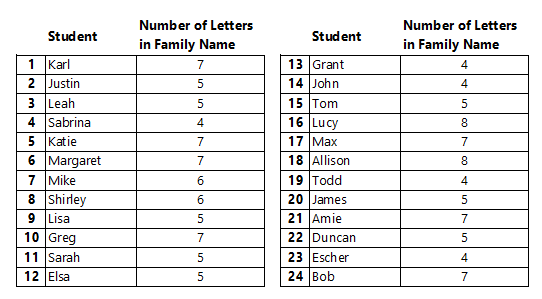A.1.1.2 Numerical Data

Number of letters in the family (last) name

Below is a list of students in a class and the number of letters they have in their family (last) name:On average, how many letters are in the family (last) names for students in this class?

Numerical Data The data in the table above is numerical because it is numbers. Other questions that would result in numerical data are: "On average, how many texts do students in this class send each day?" "On average, how many movies did students in this class watch this month?" Write your own question that would result in numerical data: